Optional (x_^n_.), (x_+y_.), (x_*y_.)

Optional (_.)  is used to indicate that a default value should be used of a
value isn't provided.  The feature can only be used with heads that have
assigned values for either Default[h], Default[h, n], or Default[h, n, i].
The only heads that have built-in default values are Plus, Times, Power, and
they are each demonstrated below.

Example using (x_^n_.)

In the first example (f) tells us the base and exponent of (s^n).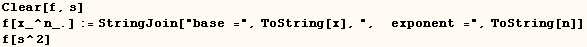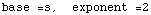The next cell (s) matches the pattern (x_^n_.) and the pattern matcher uses
the default exponent of (1).  The use of (n_.) instead of (n_) indicates that
the default value should be used if one is not provided explicitly.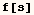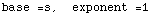Example using (x_+y_.)

In the next example (s) matches the pattern (x_+y_.) and the pattern matcher
uses the default value of (0) for (y_.) when only a single term is provided.
Because Plus has the Flat attribute the pattern matcher recognizez that
(s1+s2+s3+s4)  is a case of  Plus[s1, Plus[s2,s3,s4] ].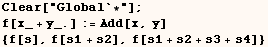Next we see that the rule is stored as a definition for  f[ y_. + x_ ]
instead of a definition for  f[ x_ + y_. ]  as entered.  I suppose that
explains why (y) is matched with (s1) in (s1+s2+s3+s4).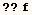Global`f

 f[y_. + x_] := Add[x, y]

Example using (x_*y_.)

In the next example (s) matches the pattern (x_*y_.) and the pattern matcher
uses the default value of (1) for (y_.) when only a single factor is
provided.  Because Times has the Flat attribute the pattern matcher
recognizez that (s1  s2  s3  s4)  is a case of  Times[s1, Times[s2, s3, s4]
].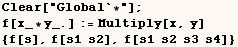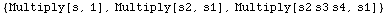Next we see that the rule is stored as a definition for  f[ (y_.)  x_ ]
instead of a definition for  f[ x_ (y_.) ]  as entered.  I suppose that
explains why (y) is matched with (s1) in

(s1  s2  s3  s4).Global`f

 f[y_. x_] := Multiply[x, y]

Other applications (rarely used)

You can also use arguments like (y_.) as arguments for functions other  than Power, Plus, Times, but only if you first assign values via Default[f],  Default[f,i] or Default[f,i,n].  To see examples of this goto the section on  Default.In the next cell the default value (z) is used when (f) is give a single
argument.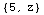Created by Mathematica  (May 16, 2004)

Back to Ted’s Tricks index page